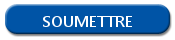# Type and structure of an object in R

## Structure of an object in R

Sometimes, we have to identify the type of the objects that we use in R. It can be useful for unit testing, to check if our data can be processed.

Thankfully, R have native advanced features to validate data format. Here are some essential features for object analysis in R.

## Get the type of an object in R

Else if the R language is tolerant, sometimes we need to get the type of an object. For example, for a data frame, here is the result that we can obtain.

```class(myDataframe)
 "data.frame"```

The class() function return a response as a character type that we can use on our scripts.

## Length of an object in R

The length of an object can be used to find the number of repetitions on a loop. You just have to use the length() function. Here is an example:

```length(myList)
 228```

The length() function return an integer with the number of elements on our list. We can use the result of this function on our script.

## Object name in R

In R, your objects can have their own name. For example, on a data frame or on a list, the elements can be identified by a name or an ID. To verify the name of an element, we can use the names() function. Here is an example:

```names(myDataframe)
 "myID" "First name" "Last name" "Age" "City"```

This function return a character vector that you can use on your R scripts.